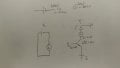# Does a power source always supplies at its rated output voltage?

Thread Starter

#### mannok

Joined Apr 5, 2020
9Consider the above 2 circuits: X and Y. Both of them have same Power Supply and Load. X is a simple circuit while Y is a circuit made with NPN BJT.

Assume the Power Supply is rated for 5V and 0.1A at maximum, the Load is rated for 0.1A@3V.

For schematic X, seems that the Load will draw only 0.1A from the Power Source and voltage dropped at the Load would be 3V. However, there is still 2V left. Where is that 2V gone? Or the Power Source would source only 3V in this case?

My question for Schematic Y is quite similar with X. Assume the BJT is in saturation mode, does the power supply source 5V and Load takes 3V while Vce takes 2V? Or the Power Source in such case would only source 3V?

Thanks in advance.

#### Papabravo

Joined Feb 24, 2006
15,562
A power supply will supply whatever current the load requires until that current exceeds the capability of the supply. At that point the voltage may drop, or the supply may shut down, or...pick a behavior. A battery, for example will keep dropping the voltage and try to supply whatever voltage is available after overcoming the source impedance. At some point the current flow will cease, and the battery dies.

Last edited:
Thread Starter

#### mannok

Joined Apr 5, 2020
9
A power supply will supply whatever current the load requires until that current exceeds the capability of the supply. At that point the voltage may drop, or the supply may shut down, or...pick a behavior. A battery, for example will keep dropping the voltage and try to supply whatever voltage is available after overcoming the source impedance. At some point the current flow will cease, and the battery dies.
So do you mean that the Power Supply will only source 3V in schematic Y and Vce would be 0?

#### Papabravo

Joined Feb 24, 2006
15,562
No the power supply will source 5V at 0.1 Amperes. If the load L takes 0.1 Amperes, and drops 3 Volts then the transistor, if it is on, and can sink 0.1 Amperes will drop 2 Volts across the CE junction.

You seem to be thinking about things in the wrong way. Instead of thinking about a load being rated @ 3V and 0.1 Amperes, you could also say that it was a 30Ω resistor and could dissipate up to say 1 watt. In the case you mentioned of 0.1 amperes going through the load and dropping 3 Volts the power being dissipated would be 300 milliwatts. Don't you think it is easier to work with one number rather than two?

It would also be reasonable to ask what would happen if you replaced the 30Ω resistor with a 15Ω resistor, and kept every thing else the same. The answer would depend on how the power source behaves when you ask it to do more than it was designed to do, and there is no single answer.

Last edited:
Thread Starter

#### mannok

Joined Apr 5, 2020
9
@Papabravo Thank you for getting me clear.

Seems make sense in thinking the Load as a resistor, my last question is, lets put a 30Ω Resistor instead of Load into both schematic X and Y.

For X, according to Ohm's Law, the battery will source only 3V as the maximum source current is 0.1A .

However, for Y, how come the case is different with X? Why does the Power Supply source 5V, the Resistor and CE junction drop 3V and 2V respectively?
Why the case would not be the Power Supply source 3V, the Resistor drop 3V and no voltage drop at CE junction as the BJT is in saturation mode?

#### Papabravo

Joined Feb 24, 2006
15,562
@Papabravo Thank you for getting me clear.

Seems make sense in thinking the Load as a resistor, my last question is, lets put a 30Ω Resistor instead of Load into both schematic X and Y.

For X, according to Ohm's Law, the battery will source only 3V as the maximum source current is 0.1A .

However, for Y, how come the case is different with X? Why does the Power Supply source 5V, the Resistor and CE junction drop 3V and 2V respectively?
Why the case would not be the Power Supply source 3V, the Resistor drop 3V and no voltage drop at CE junction as the BJT is in saturation mode?
At the top of your drawing you show the symbol for the power source and label it 5V @ 0.1 Amperes. Why are you changing it between the two schematics?
If the power source is providing current to the resistor and the transistor, then whatever current is flowing, 3 volts are dropped, because that is all there is, and the voltage across the transistor will be 0 (unlikely in practice). If the transistor is not on, then the voltage drop across the resistor will be 0.

Similar threads